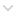Wellcome!

Question 1. Asked on :30 September 2019:10:13:55 AM

Area of Circle is ......

-Added by Akki chauhan General Knowledge » Maths

Nishant Verma
The formula to find a circle's area π (radius)2 usually expressed as π⋅r2 where r is the radius of a circle. The area of a circle is all the space inside a circle's circumference

-Answered by Nishant Verma On 08 October 2019:09:26:11 AM

Question 2. Asked on :07 September 2019:08:51:52 AM

What is full form of HCF

-Added by Sohail alam Mathematics » Maths

Akki chauhan
Highest common factor.

-Answered by Akki chauhan On 13 September 2019:09:38:11 AM

Question 3. Asked on :02 September 2019:03:04:03 PM

Q what is the area of square when side is 90cm?

-Added by Shruti Srivastva Mathematics » Maths

Shruti Srivastva
8100 cm sq.

-Answered by Shruti Srivastva On 02 September 2019:03:06:18 PM

Question 4. Asked on :30 August 2019:03:52:44 PM

write the formula of C.I.?

-Added by Suman Kumari Mathematics » Maths

Nishant Verma
CI formula
P = the principal amount. r = the annual interest rate (decimal) n = the number of times that interest is compounded per unit t. t = the time the money is invested or borrowed for.

-Answered by Nishant Verma On 30 August 2019:03:54:16 PM

Question 5. Asked on :25 August 2019:03:25:31 PM

Find the next  number in the sequence 6,24,60,120.......

-Added by Akki chauhan Mathematics » Maths

priyanshu kumar

336.

-Answered by priyanshu kumar On 20 October 2019:04:14:05 PM

Question 6. Asked on :25 August 2019:03:23:16 PM

Find the next number in the sequence 0,2,24,252......Please give right answer other wise dont touch this question.

-Added by Akki chauhan Mathematics » Maths

Shivang Gupta

0,2,24,252 Adding 1,2,3,4 n 5 respectively, 0+1=1 2+2=4 24+3=27 252+4=256 We get, 1,4,27,256 Now, 1=1^1 4=2^2 27=3^3 256=4^4 So, next no. = 5^5 - 5= 3120 :)-Answered by Shivang Gupta On 25 August 2019:03:38:16 PM

Question 7. Asked on :13 May 2019:10:29:43 AM

7 और  9 के बीच 10 परिमेय संख्या लिखे ?

-Added by ravi varma Mathematics » Maths

Himanshi Verma

The rational numbers between 7 and 9 are 78, 79, 80, 81, 82, 83, 84, 85, 86, 87, 88, 90.

-Answered by Himanshi Verma On 14 May 2019:11:11:27 AM

Question 8. Asked on :08 May 2019:10:19:35 AM

x का मान  ज्ञात कीजिए  2x-1=14-x?

-Added by ravi varma Mathematics » Maths

Jyoti Srivastva

2x-1=14-x

2x+x=14+1

3x=15

x=15/3

x=5

-Answered by Jyoti Srivastva On 07 July 2019:10:05:52 PM

Question 9. Asked on :08 May 2019:10:15:06 AM

4z+3=6+2z

-Added by ravi varma Mathematics » Maths

Jyoti Srivastva

4z+3=6+2z

4z-2z=6-3

2z=3

z=3/2

-Answered by Jyoti Srivastva On 07 July 2019:10:11:51 PM

Question 10. Asked on :01 March 2019:03:26:35 PM

How to prove Pythagorus theorem .

-Added by Akki chauhan Mathematics » Maths

Himanshi Verma

ABC is a right angle triangle at B and BD is a perpendicular from B on to AC, the diagonal.

Triangles ABC and BDC are similar, as:

. angle DBC = 90 - angle C = angle A

. angle ABC = angle BDC = 90

Ratios of corresponding sides are equal.

AB / BD = BC / DC = AC / BC

So we get  BC² = AC * DC     --- (1)

Triangle ABC amd ADB are similar, as:

. angle DBA = 90 - (90 - C) = angle C

. angle ABC = 90 = angle ADB

so ratios of corresponding sides are equal

AB / AD = BC / BD = AC / AB

So we get  AB² = AD * AC  --- (2)

Add (1) and (2) to get:

AB² + BC² = AC * ( AD + DC) = AC * AC = AC²

So the theorem is proved.

-Answered by Himanshi Verma On 01 March 2019:03:38:26 PM

Question 11. Asked on :20 January 2019:10:13:42 AM

cotΦ-1/sinΦ=1

-Added by Rohit Rajput Mathematics » Maths

Master Purushottam

Cscϕ/secϕ = (1+cotϕ)/(1+tanϕ)

LHS = (1+cotϕ)/(1+tanϕ)

where cotϕ=cosϕ/sinϕ and tanϕ = sinϕ/cosϕ

= (1+(cosϕ/sinϕ))/(1+(sinϕ/cosϕ))

= ((cosϕ+sinϕ)/sinϕ)/((cosϕ+sinϕ)/cosϕ)

= cosϕ/sinϕ

wkt cosϕ=1/secϕ

and sinϕ=1/cosecϕ

= cscϕ/secϕ

LHS = RHS

Hence Proved

-Answered by Master Purushottam On 24 August 2019:12:17:05 AM

Question 12. Asked on :18 January 2019:05:21:24 PM

Write the formula of cylinder open from the top ?

-Added by khushi chauhan Mathematics » Maths

Master Purushottam

Total surface area= 2πr(r+h) -πr2

curved surface area = 2πrh+πr2

-Answered by Master Purushottam On 24 August 2019:12:15:48 AM

You can see here all the solutions of this question by various user for NCERT Solutions. We hope this try will help you in your study and performance.

This Solution may be usefull for your practice and CBSE Exams or All label exams of secondory examination. These solutions or answers are user based solution which may be or not may be by expert but you have to use this at your own understanding of your syllabus.

What do you have in your Mind....

* Now You can earn points on every asked question and Answer by you. This points make you a valuable user on this forum. This facility is only available for registered user and educators.

Do you have a question to ask?

User Earned Point: Select

All Tags by Subjects:

Science (2235)
History (278)
Geography (310)
Economics (258)
Political Science (96)
Mathematics (201)
General Knowledge (5686)
Biology (94)
Physical Education (20)
Chemistry (118)
Civics (114)
Home Science (12)
Sociology (9)
Hindi (45)
English (259)
Physics (1435)
Other (97)
Accountancy (378)